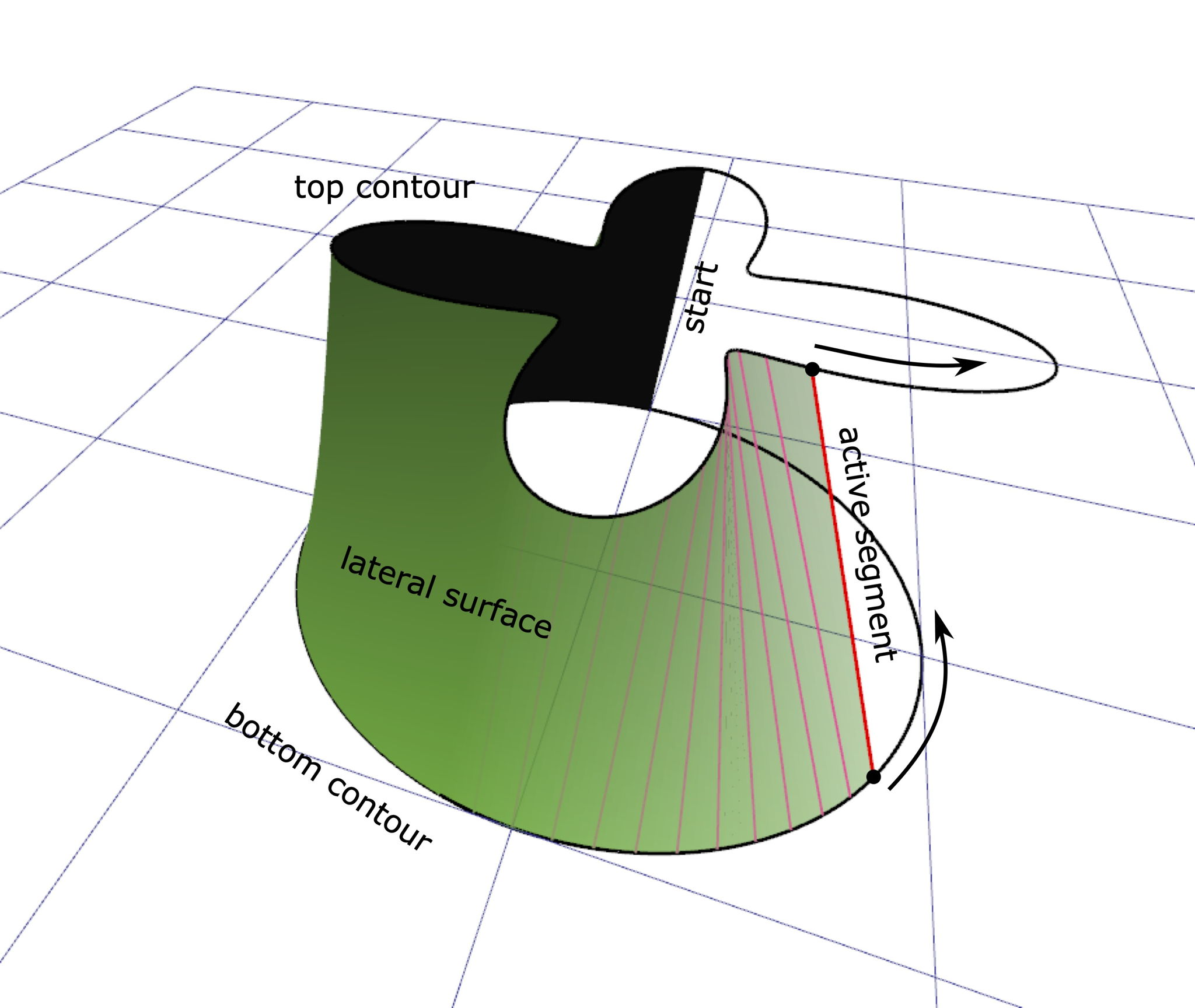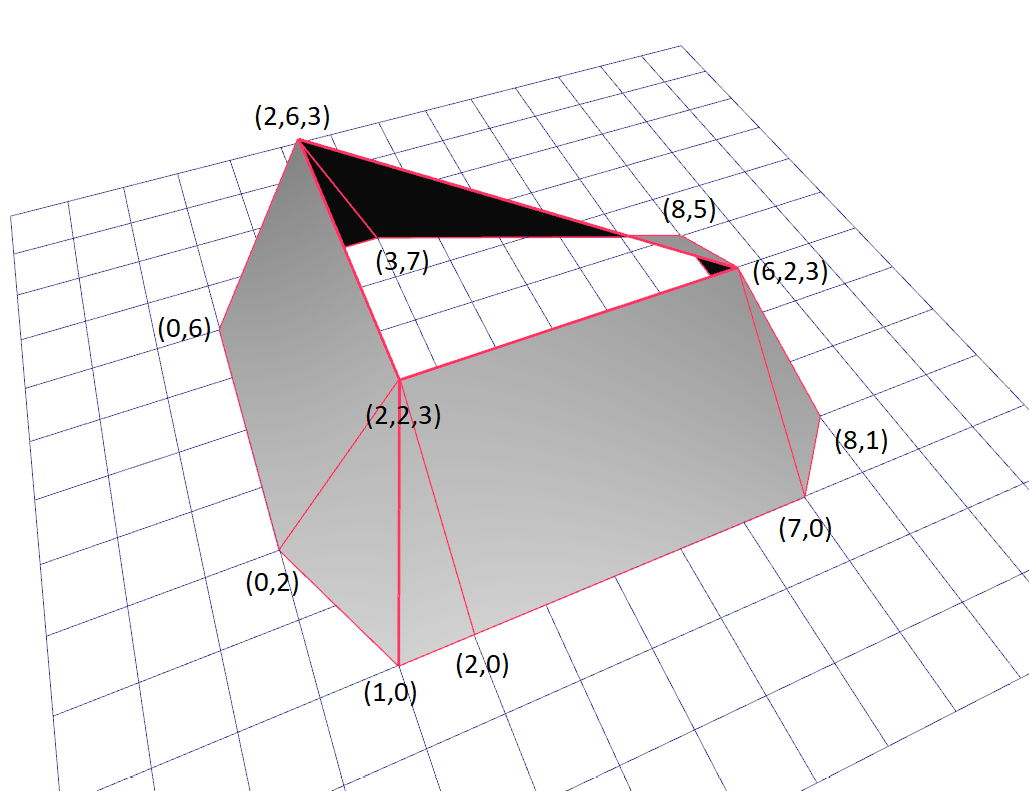시간 제한메모리 제한제출정답맞힌 사람정답 비율
3 초 (추가 시간 없음) 256 MB111100.000%

## 문제

A popular architectural CAD program has an operation to create a solid body called "Blend". This operation takes two contours lying in parallel planes and builds a body between these contours. The area bounded by each of the contours in its plane becomes a top or bottom face of the body, and a set of lateral faces is constructed between the two contours.

The lateral surface is built in the following manner (see figure 1 below samples). On each of the contours, a start point is chosen. Each contour has a predefined orientation, i.e. a direction of traversal. The selected start points are joined by an active segment (which is a straight line segment). Next, the ends of the active segment are moved along the contours: the end on the bottom contour is moved along the bottom contour, and the end on the top contour is moved along the top contour. As the active segment moves, it sweeps the lateral surface. To obtain the complete lateral surface of the resulting body, each of the endpoints must go one full turn around the corresponding contour. It is forbidden to move an end of the active segment against the chosen direction of contour transversal.

For the purpose of this problem, assume that each contour is a closed polyline consisting of line segments. Note that the rules described above are ambiguous: you can build all sorts of lateral surfaces for the contours by varying how fast you move the ends of the active segment. To resolve the ambiguity, additional rules are introduced. When one end of the active segment is at a vertex of the polyline, the other end must also be at a vertex of the other polyline. Such positions of the active segment define the lateral edges of the created body, and the parts of the lateral surface between consecutive lateral edges are called lateral faces.

Hence each lateral face of the body must either join a segment of one of the polylines with a vertex of the other polyine (a triangle), or join a segment of one of the polylines with a segment on the other polyline (a quadrangle). The quadrangle can even be curvilinear: in this case, its precise geometry depends on the relative speed of the ends of the active segment. In reality, these speeds are also regulated, but this is irrelevant for this problem. Ultimately, the resulting body is strictly defined if we select which lateral edges must be built, i.e. how the vertices of the given polylines must be matched.

Generally, this is defined by the engineer --- the CAD software user. For simplicity, the program provides a default matching. It is chosen in such a way that the total length of all the lateral edges is minimal possible. Write a program that will calculate this default matching based on the provided input contours.

Note that this description does not tell anything about self-intersections of the lateral surface. Naturally, if these problems do occur, the body will not be a solid body, strictly speaking. Such anomalies are possible and allowed with the "Blend" operation, including the case when lateral edges are chosen by default. The discovery and correction of these problems is done with completely different algorithms.

## 입력

The first line contains three integers: $M$ --- number of vertices in the bottom polyline, $N$ --- number of vertices in the top polyline, and $H$ --- height of the body ($3 \le M, N \le 300$, $1 \le H \le 10^6$).

The following $M$ lines define the coordinates of the vertices of the bottom polyline. Each line contains two integers: x- and y- are the coordinates of the vertex. Vertices are listed according to the selected direction of traversal of the polyline; the last defined vertex is followed by the first defined vertex. The remaining $N$ lines contain the coordinates of the top polyline in the same format.

All coordinates do not exceed $10^6$ in absolute value. The bottom polyline lies in the plane $z = 0$, and the top polyline lies in the plane $z = H$. All vertices on every polyline are distinct. Self-intersections are allowed.

## 출력

In the first line of the output file, print a real number $A$ --- the total length of the lateral edges in your solution, and an integer $K$ --- the number of lateral edges ($\max(M,N) \le K \le M+N$).

In the remaining $K$ lines, print the lateral edges in the order as they are sweeped by the active segment. You can start from any lateral edge. For each lateral edge, print a separate line with two integers $i$ and $j$ --- the indices of the vertices joined by the edge the in bottom and top polylines, respectively ($1 \le i \le M$, $1 \le j \le N$).

The absolute or relative deviation of the value $A$ from the optimal one must not exceed $10^{-9}$.

## 예제 입력 1

3 3 1
0 0
2 0
1 1
3 -1
1 2
-1 -1


## 예제 출력 1

4.878315177510850 3
1 3
2 1
3 2


## 예제 입력 2

9 3 3
0 2
1 0
2 0
7 0
8 1
8 5
3 7
2 7
0 6
6 2
2 6
2 2


## 예제 출력 2

33.210944197060996 9
1 3
2 3
3 3
4 1
5 1
6 1
7 2
8 2
9 2


## 노트Figure 1: Lateral surface sweeped by the active segment.Figure 2: Second sample: lateral edges and faces.